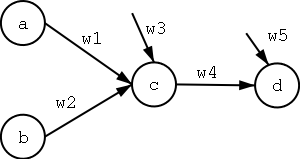# CSCE 883: Test 1

## Name:___________________________________________________

Show your work for every question but make clear which one is the final answer by drawing a box around it. Remember to copy the question number to your answer booklet.

1. [5 points] You are asked to write a program that learns to categorize news articles into a set of categories such as: sports, entertainment, politics, business, humor, editorial, etc. Describe how you would start this task. Specifically, what is your
1. performance measure,
2. training experience,
3. and target function to be learned.
2. [5 points] What is the difference between a concept, a target function, and a target function representation. Give an example for all three. The example cannot be a board game.
3. [5 points] You can tell someone: "Here is a book on Java. Read it and you will learn everything about Java." Why is this not a well-posed learning problem?
4. [5 points] Why do we need the Inductive Learning Hypothesis? Specifically, what happens to our learning algorithms when it is not true?
5. [5 points] Show the partial ordering for the following hypothesis from general to specific. They use the notation from Chapter 2.
1. <Red, Hot, Dark, Moody>
2. <White, ?, Light, Moody>
3. <?, Hot, Dark, ?>
4. <White, ?, Dark, Moody>
5. <Red, ?, Dark, ?>
6. <Blue, ?, ?, ?>
7. <Blue, Cold, Sunny, Calm>
6. [10 points] Give the general and specific hypothesis that represent (i.e. bound) the version space VH,D where H is the set of all hypothesis represented by an ordered triple of three argument values and all values are taken from the set {1,2,3,4}. D is the set:
<2,3,4>, +
<1,3,4>, +
<4,3,4>, -
<1,1,1>, -
<2,4,2>, +
<3,1,3>, +
7. [5 points] Consider a learning problem where the instances are represented by three different attributes, each of which can have four different values. The hypothesis are boolean functions of the form
(x1=v1 ∨ x2=v2) ∧ (x3=v3 ∨ x4=v4)
where xi is one of the attributes and vi is one of the values. Give a tight upper bound for the size of the hypothesis space? If your bound is not the exact size of the hypothesis space, explain which are the missing cases.
8. [10 points] Consider the following set of training examples
Instance Classification a1 a2
1 - T T
2 + T F
3 + F F
4 - F T

What is the entropy of this collection of training examples with respect to the target function classification?
9. [5 points] Give decision trees (one for each) that represent:
1. (A ∨ B) ∧ (C ∨ D)
2. (A ∧ B) ∨ (B ∧ C ∧ A) ∨ (A ∧ F) ∨ (B ∧ C)
10. [10 points] If we wanted to use decision tree learning with examples that had one continuous attribute (temperature) with values:
Temperature Classification
88 +
92 -
94 -
95 +
99 +
100 -
110 +
What is the attribute we would add, according to the algorithm given in section 3.7.2?
11. [10 points] I have a box with 100 marbles. I will be randomly choosing one and writing its color down on a list, which I then want to tell you about. What is the minimum number of bits that I will need in order to encode the information contained in this list? Assume that we both know that the box contains 80 black, 15 white, and 5 red marbles.
12. [10 points] What are the weights w0, w1, and w2 for a perceptron that divides the plane with the line y = (1/4)x + 1/2. Assume that input x1 (which is matched to w0) is mapped to the x-axis and x2 is mapped to the y-axis.
13. [5 points] Why did we need multi-layer networks? That is, why are perceptrons not enough?
14. [10 points] Consider the two-layer neural networkwhere a,b are the inputs, c is the hidden units, d is the output unit, w1,w2,w4 are link weights, and w3,w5 are thresholds. Initialize all the weights to .1. Assume a learning rate of .2. Using the training examples
a b d
0 1 1
1 1 0
give the weight values after the first two training iterations of the Backpropagation algorithm.

Jose M. Vidal